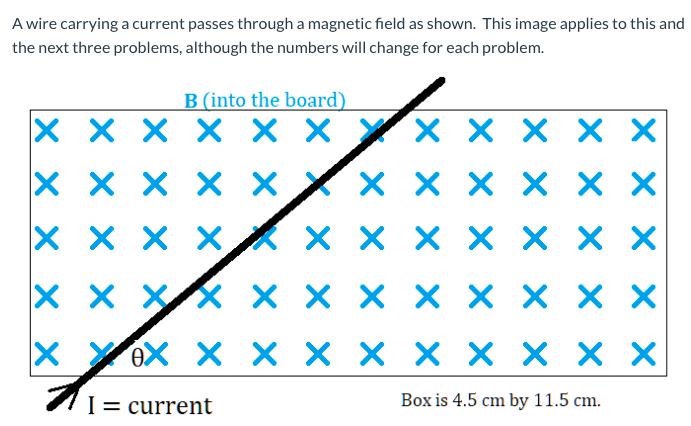# B (into the board) X x X X × × YX × × × X x x x x x × × x × × x x x XX X x × x X × × x X YeX X × X X X × X X X I = current Box is 4.5 cm by 11.5 cm.

Question

What is the magnitude of the magnetic force?  (Answer in Newtons.)   (B = 0.044 T, θ = 32°, I = 4.31 A)help_outlineImage TranscriptioncloseB (into the board) X x X X × × YX × × × X x x x x x × × x × × x x x XX X x × x X × × x X YeX X × X X X × X X X I = current Box is 4.5 cm by 11.5 cm. fullscreen

### Want to see this answer and more?

Experts are waiting 24/7 to provide step-by-step solutions in as fast as 30 minutes!*

*Response times may vary by subject and question complexity. Median response time is 34 minutes for paid subscribers and may be longer for promotional offers.
Tagged in
Science
Physics

### Electric Charges and Fields